Interference

When two groups of waves (called wave trains) meet and overlap they interfere with each other. The resulting amplitude will depend on the amplitudes of both the waves at that point.

If the crest of one wave meets the crest of the other the waves are said to be in phase and the resulting intensity will be large. This is known as constructive interference. If the crest of one wave meets the trough of the other (and the waves are of equal amplitude) they are said to be out of phase by p then the resulting intensity will be zero. This is known as destructive interference.

This phase difference may be produced by allowing the two sets of waves to travel different distances - this difference in distance of travel is called the path difference
There will be many intermediate conditions between these two extremes that will give a small variation in intensity but we will confine ourselves to total constructive or total destructive interference for the moment.

The diagrams in Figure 1 below show two waves of equal amplitudes with different phase and path differences between them. The first pair have a path difference of a whole number of wavelengths, including zero. The second pair have a phase difference of p or 180o and a path difference of an odd number of half-wavelengths.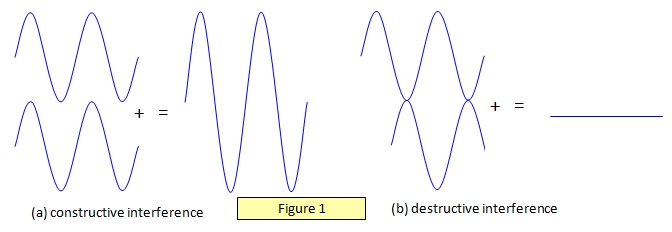Figure 1(a) shows destructive interference and Figure 1(b) constructive interference.

To obtain a static interference pattern at a point (that is, one that is constant with time) we must have:
(a) two sources of the same wavelength, and
(b) two sources which have a constant phase difference between them.
Sources with synchronised phase changes between them are called coherent sources and those with random phase changes are called incoherent sources.

This condition is met by two speakers connected to a signal generator because the sound waves that they emit are continuous – there are no breaks in the waves.

However two separate light sources cannot be used as sources for a static interference pattern because although they may be monochromatic the light from them is emitted in a random series of pulses of around 10-8 s duration. The phase difference that may exist between one pair of pulses emitted from the source may well be quite different from that between the next pair of pulses (Figure 2).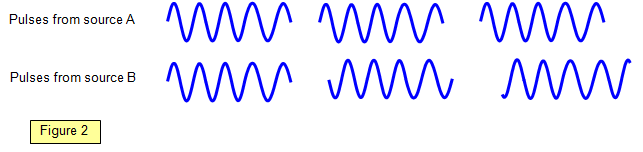Therefore although an interference pattern still occurs, it changes so rapidly that you get the impression of uniform illumination. Another problem is that the atoms emitting the light may collide with each other so producing phase changes within one individual photon. We must therefore use one light source and split the waves from it into two in some way.

There are two ways of doing this:
(a) division of amplitude, where the amplitude at all points along the wavefront is divided between the two secondary waves, and
(b) division of wavefront, where the original wave-front is divided in two, half of it forming each of the secondary waves.
However, the length of each pulse limits the path difference that we may obtain between even these two waves from the same source. Since the pulses are only about 10-8 s long the maximum path difference is 3 m, although in practice good results are only obtained with shorter path differences than this.

Interference between two waves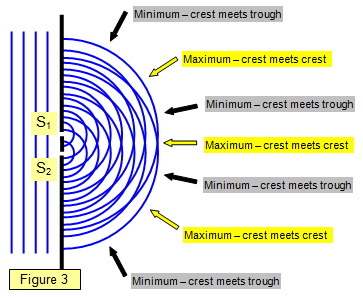The diagrams in Figure 3-7 show two sources S1 and S2 emitting waves - they could be light, sound or microwaves.
The plan view of the waves in Figure 3 shows waves coming from two slits and interfering with each other. The lines along which the path differences will give maxima or minima.

This type of arrangement is like that produced in a ripple tank or in the double slits experiment with light (see later).

It should be realised that between the maxima and minima the intensity will change gradually from one extreme to the other.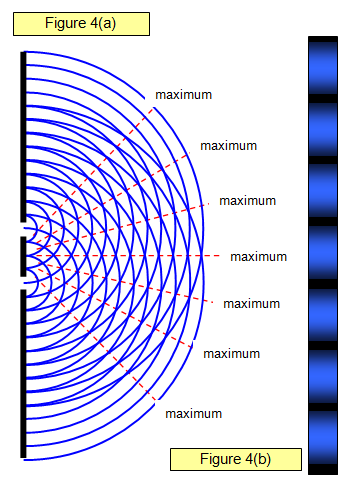Figure 4(a) shows light interfering as it passes through two slits. In Figure 4(b) the appearance of the interference pattern on a screen placed in the path of the beam is shown.

You can see the maxima and minima and the way in which the intensity changes from one to the other.

Changing the wavelength of the light (its wavelength), the separation of the slits or the distance of the slits from the screen will all give changes in the separation of the maxima in the interference pattern.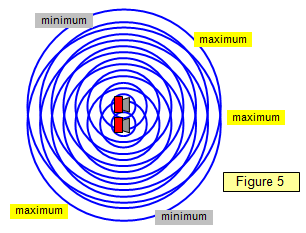Figure 5 shows the interference effects of two speakers. The sound waves spread out all round the speakers and a static interference pattern is formed. (Not all the maxima and minima are labelled).

You can hear this by setting up two speakers in the lab connected to one signal generator and then simply walking round the room. You will hear the sound go from loud to soft as you pass from maximum to minimum. (A frequency of around 400 Hz is suitable).

In Figures 6 and 7 you can see that at the different points on the screen the waves from S1 have travelled a different distance from those from S2. In Figure 6 the path difference is zero, in Figure 7 it is half a wavelength.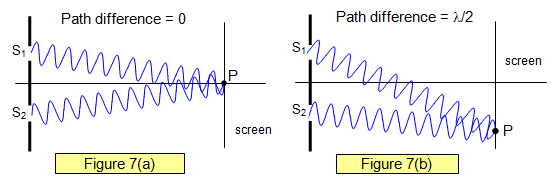Path length in a material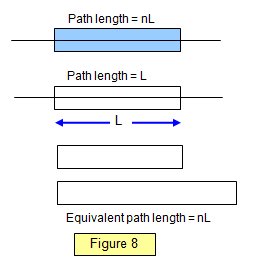When light passes through a material of refractive index n it is slowed down, its velocity in the material being 1/n times that in a vacuum.
For example, the velocity of light in glass is about 2.0x108 ms-1 compared with about 3.00x108 ms-1 in a vacuum.

The time light takes to pass through a given length of the material is therefore n times that which it takes to pass through the same length of air.

The path length in a material of length L and refractive index n is therefore nL (Figure 8). If one part of a light beam travels a distance L in air and the other a distance L in the material then a path difference will exist between them of L(n - 1) and if the two beams are made to overlap an interference pattern will result.

Example problem
Calculate the path difference formed between two beams of yellow light (wavelength = 600 nm), one of which passes through 1 m of air (refractive index 1.000298) and the other that passes through 1 m of free space (a vacuum).

Path difference = 1(1.000298 - 1) = 0.000298 m = 0.298 mm = 0.298/0.0006 = 500 wavelengths

A VERSION IN WORD IS AVAILABLE ON THE SCHOOLPHYSICS USB